# Adaptive Terminal Synergetic Synchronization of Hyperchaotic Systems

Adaptive Terminal Synergetic Synchronization of Hyperchaotic Systems

Electrical Engineering Department, University Ferhat Abbas Setif 1, Setif 19000, Algeria

QUERE Laboratory University Ferhat Abbas Setif 1, Setif 19000, Algeria

Electrical Engineering Department, University Mohammed El Bachir El-Ibrahimi BordjBou-Arreridj, BordjBou-Arreridj 34000, Algeria

Corresponding Author Email:
Page:
789-795
|
DOI:
https://doi.org/10.18280/jesa.540515
26 October 2020
|
Accepted:
15 July 2021
|
Published:
31 October 2021
| Citation

OPEN ACCESS

Abstract:

This research paper introduces an adaptive terminal synergetic nonlinear control. This control aims at synchronizing two hyperchaotic Zhou systems. Thus, the adaptive terminal synergetic control’s synthesis is applied to synchronize a hyperchaotic i.e., slave system with unknown parameters with another hyperchaotic i.e., master system. Accordingly, simulation results of each system in different initial conditions reveal significant convergence. Moreover, the findings proved stability and robustness of the suggested scheme using Lyapunov stability theory.

Keywords:

hyperchaotic Zhou system, terminal synergetic, synchronization, Lyapunov

1. Introduction

Chaos theory and chaotic systems are deterministic, irregular, and aperiodic with unpredictable behavior having an extra-sensitive dependence on initial conditions . Hence, chaotic systems are generally defined as a set of two or three autonomous nonlinear equations. Accordingly, a three-dimensional chaotic system includes one positive, one zero, and one negative Lyapunov exponents. Thus, if a system has more than three states and displays chaotic behavior, then it is referred to as a hyperchaotic system , that was first discovered by Rössler . For instance, among the classical common systems are, Lorentz system , hyperchaotic Lü system , Chua’s circuit , and Qi system ; in addition, the recent hyperchaotic systems are Dadras system , hyperchaotic Vaidyanathan systems , hyperchaotic Sampath system , and hyperchaotic Pham system . On the other hand, a four-dimensional hyperchaotic system contains one more positive Lyapunov exponent. It has complex dynamics and characteristics than chaotic systems; therefore, this type of dynamics has miscellaneous applications in engineering such as secure communications [12, 13], cryptosystems , encryption [15, 16], and electrical circuits [17, 18].

Furthermore, in the last two decades, synchronization of chaotic and hyperchaotic systems has been a crucial topic of research, it is required when a chaotic system drives another chaotic system reaching asymptotically zero error between master and slave systems states. Among synchronization types studied recently in the literature are: complete synchronization , anti-synchronization [20, 21], hybrid synchronization [22, 23], lag synchronization , phase synchronization , anti-phase synchronization , generalized synchronization , projective synchronization , generalized projective synchronization [29-31], etc.

In the same vein, since the discovery of chaos synchronization applications, various control techniques and methods have been developed, such as active control method  can be used when the system parameters are known, adaptive control method [31, 33] is applied when the system parameters are unknown., nonlinear feedback , backstepping control  and sliding mode control .

Similar to sliding mode (SMC) but without chattering, the synergetic technique is a robust approach which doesn’t need linearization with no discontinuous term in its control law is thus more suited for real-time implementation. As in sliding mode control upon reaching the equilibrium point, the system dynamics remain insensitive to a class of parameter deviations and disturbances. A variety of successful applications of this approach exist such as a battery charging system , a power system stabilizer [38, 39], a quadrotor helicopter system , and DC_DC power converter control [41, 42].

The goal considered in this paper, is to force the master-slave hyperchaotic systems to be synchronized even if they have differential initial conditions. Simulation results show that the proposed controller effectively drives the slave system in spite of different initial conditions. In this paper, Section 2 introduces the hyperchaotic Zhou system while Section 3 covers the main results for the adaptive terminal synchronization of the identical Zhou systems with unknown parameters using terminal synergetic control. Finally, the concluding remarks are given in Section 4.

2. System Description

In 2009, hyperchaotic Zhou system  is described by a fourth order model.

$\dot{x}_{1}=a\left(x_{2}-x_{1}\right)+x_{4}$

$\dot{x}_{2}=c x_{2}-x_{1} x_{3}$

$\dot{x}_{3}=-b x_{3}+x_{1} x_{2}$

$\dot{x}_{4}=d x_{1}+0.5 x_{2} x_{3}$     (1)

x1, x2, x3 and x4 represent the system variables, and a, b, cand d are system parameters. Hyperchaotic comportment for (1) can be observed for:

a=35, b=3, c=12, 0<d<34.8     (2)

Using (2), the system linearization matrix at the equilibrium point E0=[0 0 0] is obtained as:

$A=\left[\begin{array}{cccc}-a & a & 0 & 1 \\ 0 & c & 0 & 0 \\ 0 & 0 & -b & 0 \\ d & 0 & 0 & 0\end{array}\right]$    (3)

Eigenvalues of A are:

$\lambda_{1}=c, \lambda_{2}=-b, \lambda_{3}=\frac{-a+\sqrt{a^{2}+4 d}}{2}$

$\lambda_{4}=\frac{-a-\sqrt{a^{2}+4 d}}{2}$     (4)

It is obvious that system (1) is unstable, λ1being positive.

In the simulation study, the values of a, b, c are as given in (2) and the value of d is chosen as d=1.Projections of different attractors are shown in Figure 1. This figure shows the behavior chaotic of Zhou system.

## 11.png## 12.png## 13.png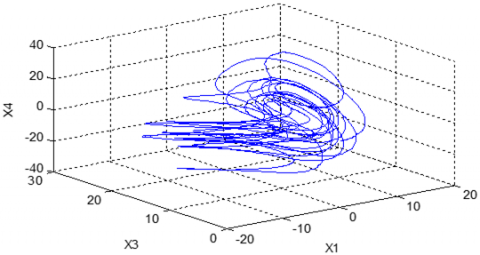## 14.png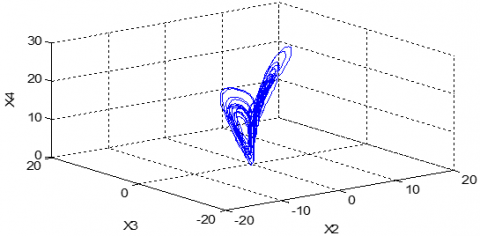Figure 1. Hyperchaotic Zhou system phase plane portrait

3. Synergetic Control

Synergetic control is a robust nonlinear approach very similar to sliding mode control technique; it relies on a suitable macro-variable choice which comprises variables of interest and a desired performance based constraint.

Synthesis of the controller begins with a choice of a function of two or more state variables called the macro-variable (5):

$\dot{x}(t)=f(x, u, t)$     (5)

x is the system state vector and u the control law.

Synergetic control includes the choice of a function of two or more system variables called the macro-variable as in (6):

$\Psi=\Psi(x, t)$     (6)

The synergetic control goal is to drive the system to a chosen manifold.

$\dot{\Psi}=\frac{d \Psi}{d x} \dot{x}$     (7)

T represents the desired speed convergence to the selected manifold.

$\dot{\Psi}=\frac{d \Psi}{d x} \dot{x}$     (8)

Substituting (9) and (6) into (8) gives:

$\mathrm{T} \frac{d \Psi}{d x} f(x, u, t)+\Psi=0$     (9)

Solving (9) for u leads to (10):

$u=g(x, \Psi(x, t), \mathrm{T}, t)$    (10)

4. Adaptive Synchronization Using Terminal Synergetic Control

An adaptive scheme will be used in this section in conjunction with a terminal synergetic approach to provide a robust control law for globally synchronizing identical hyperchaotic Zhou systems with unknown parameters.

Letting the master system be given as:

$\dot{x}_{1}=a\left(x_{2}-x_{1}\right)+x_{4}$

$\dot{x}_{2}=c x_{2}-x_{1} x_{3}$

$\dot{x}_{3}=-b x_{3}+x_{1} x_{2}$

$\dot{x}_{4}=d x_{1}+0.5 x_{2} x_{3}$      (11)

The slave system with the terminal synergetic controllers (ui) is defined as follows:

$\dot{y}_{1}=\hat{a}\left(y_{2}-y_{1}\right)+y_{4}+u_{1}$

$\dot{y}_{2}=\hat{c} y_{2}-y_{1} y_{3}+u_{2}$

$\dot{y}_{3}=-\hat{b} y_{3}+y_{1} y_{2}+u_{3}$

$\dot{y}_{4}=\hat{d} y_{1}+0.5 y_{2} y_{3}+u_{4}$      (12)

$y_{1}, y_{2}, y_{3}, y_{4}$ represent system states and $u_{1}, u_{2}, u_{3}, u_{4}$ the adaptive controls to be elaborated based on estimates $\hat{a}, \hat{b}, \hat{c}, \hat{d}$  for the unknown parameters a, b, c, d.

For the complete synchronization of the master and slave systems, the synchronization errors must rapidly reach a zero value i.e.: $\lim _{t \rightarrow \infty} e_{i}=0$. Where ei is defined by:

$e_{i}=y_{i}-x_{i}$    (13)

Thus, the synchronization errors dynamics can be described by (14):

$\dot{e}_{1}=\hat{a}\left(y_{2}-y_{1}\right)+y_{4}-a\left(x_{2}-x_{1}\right)-x_{4}+u_{1}$

$\dot{e}_{2}=\hat{c} y_{2}-y_{1} y_{3}-c x_{2}+x_{1} x_{3}+u_{2}$

$\dot{e}_{3}=-\hat{b} y_{3}+y_{1} y_{2}+b x_{3}-x_{1} x_{2}+u_{3}$

$\dot{e}_{4}=\hat{d} y_{1}+0.5 y_{2} y_{3}-d x_{1}-0.5 x_{2} x_{3}+u_{4}$     (14)

4.1 Control design

The main objective here is to design adaptive terminal synergetic controllers $\left(u_{1}, u_{2}, u_{3}, u_{4}\right)$ to synchronize the hyperchaotic systems in Eq. (12) with Eq. (11).

First, a macro-variable $\Psi$ is defined to construct a manifold for the nonlinear system to be controlled given as:

$\Psi_{i}=e_{i}+k_{i} \int_{0}^{t} e_{i}^{\frac{p_{i}}{q_{i}}}(\tau) d \tau$

$\Psi_{i}=0$     (15)

The derivative of (15) leads to:

$\dot{\Psi}_{i}=\dot{e}_{i}+k_{i} e_{i}^{\frac{p_{i}}{q_{i}}}$    （16）

where, $i=1,2,3,4$ and $k_{i}>0, p$ and $q$ are positive odd constants, such that 0< $\frac{p}{q}$ <1. Upon reaching the terminal attractor $\Psi=0$, the error system dynamics is constrained by (17):

$\dot{e}_{i}=-k_{i} e_{i}^{\frac{p_{i}}{q_{i}}}$    (17)

which may be written as (18):

$d t=-\frac{1}{k_{i}} e_{i}^{-\frac{p_{i}}{q_{i}}} d e_{i}$     (18)

Time integrating (18) $\left(e_{i}(0) \neq 0, e\left(t_{f}\right)=0\right)$ leads to the following equation:

$t_{f}=\frac{e_{i}(0)^{1-\frac{p_{i}}{q_{i}}}}{k_{i}\left(1-\frac{p_{i}}{q_{i}}\right)}$     (19)

When the system reaches the terminal synergetic mode at $t=t_{f}$, the system state error converges to zero in finite-time.

The adaptive synergetic controllers obtained are given in (20):

$u_{1}=-\hat{a}\left(e_{2}-e_{1}\right)-e_{4}-k_{1} e_{1}^{\frac{p_{1}}{q_{1}}}-\frac{\Psi_{1}}{\mathrm{~T}_{1}}$

$u_{2}=-\hat{c} e_{2}+y_{1} y_{3}-x_{1} x_{3}-k_{2} e_{2}^{\frac{p_{2}}{q_{2}}}-\frac{\Psi_{2}}{\mathrm{~T}_{2}}$

$u_{3}=\hat{b} e_{3}-y_{1} y_{2}+x_{1} x_{2}-k_{3} e_{3}^{\frac{p_{3}}{q_{3}}}-\frac{\Psi_{3}}{\mathrm{~T}_{3}}$

$u_{4}=-\hat{d} e_{1}-0.5 y_{2} y_{3}+0.5 x_{2} x_{3}-k_{4} e_{4}^{\frac{p_{4}}{q_{4}}}-\frac{\Psi_{4}}{\mathrm{~T}_{4}}$      (20)

The estimated unknown parameters are obtained using the following adaptive laws:

$\dot{\hat{a}}=-\Psi_{1}\left(x_{2}-x_{1}\right)$

$\dot{\hat{b}}=\Psi_{3} x_{3}$

$\dot{\hat{c}}=-\Psi_{2} x_{2}$

$\dot{\hat{d}}=-\Psi_{4} x_{1}$      (21)

4.2 Robust stability analysis of the controller

Lyapunov stability analysis is used to study the stability of the controlled system.

Theorem: The adaptive terminal synergetic control input law in Eq. (20) with $k_{i}>0$ and $T_{i}>0$ stabilizes the system.

Proof: A Lyapunov function candidates chosen as:

$V=\frac{1}{2} \sum_{1}^{4} \Psi_{i}^{2}+\frac{1}{2} \sum_{1}^{4} \alpha_{i}^{2}$     (22)

where the parameter estimation errors are defined as:

$\alpha_{1}=\hat{a}-a$

$\alpha_{2}=\hat{b}-b$

$\alpha_{3}=\hat{c}-c$

$\alpha_{4}=\hat{d}-d$    （23）

The derivative of the Lyapunov function gives:

$\dot{V}=\sum_{1}^{4} \Psi_{i} \dot{\Psi}_{i}+\sum_{1}^{4} \alpha_{i} \dot{\alpha}_{i}$    (24)

Using (16) and (23) in (24) results in (25)

$\dot{V}=\Psi_{1}\left[\hat{a} y_{2}-\hat{a} y_{1}+y_{4}-a x_{2}+a x_{1}-x_{4}+u_{1}+k_{1} e_{1}^{\frac{p_{1}}{q_{1}}}\right]$

$+\Psi_{2}\left[\hat{c} y_{2}-y_{1} y_{3}-c x_{2}+x_{1} x_{3}+u_{2}+k_{2} e_{2}^{\frac{p_{2}}{q_{2}}}\right]$

$+\Psi_{3}\left[-\hat{b} y_{3}+y_{1} y_{2}+b x_{3}-x_{1} x_{2}+u_{3}+k_{3} e_{3}^{\frac{p_{3}}{q_{3}}}\right]$

$+\Psi_{4}\left[\hat{d} y_{1}+0.5 y_{2} y_{3}-d x_{1}-0.5 x_{2} x_{3}+u_{4}+k_{4} e_{4}^{\frac{p_{4}}{q_{4}}}\right]$

$+\alpha_{1} \dot{\hat{a}}+\alpha_{2} \dot{\hat{b}}+\alpha_{3} \dot{\hat{c}}+\alpha_{4} \dot{\hat{d}}$      (25)

which leads after some basic mathematical manipulations to (26):

$\dot{V}=\Psi_{1}\left[u_{1}+\hat{a}\left(e_{2}-e_{1}\right)+e_{4}+k_{1} e_{1}^{\frac{p_{1}}{q_{1}}}\right]$

$+\Psi_{2}\left[u_{2}+\hat{c} e_{2}-y_{1} y_{3}+x_{1} x_{3}+k_{2} e_{2}^{\frac{p_{2}}{q_{2}}}\right]$

$+\Psi_{3}\left[u_{3}-\hat{b} e_{3}+y_{1} y_{2}-x_{1} x_{2}+k_{3} e_{3}^{\frac{p_{3}}{q_{3}}}\right]$

$+\Psi_{4}\left[\hat{d} e_{1}+0.5 y_{2} y_{3}-0.5 x_{2} x_{3}+u_{4}+k_{4} e_{4}^{\frac{p_{4}}{q_{4}}}\right]$

$+\alpha_{1}\left[\Psi_{1}\left(x_{2}-x_{1}\right)+\dot{\hat{a}}\right]+\alpha_{2}\left(\Psi_{2} x_{2}+\dot{\hat{c}}\right]$

$+\alpha_{3}\left(-\Psi_{3} x_{3}+\dot{\hat{b}}\right)+\alpha_{4}\left(\Psi_{4} x_{1}+\dot{\hat{d}}\right)$     (26)

Making use of (20), (21) in (26) permits to write:

$\dot{V}=-\frac{\Psi_{1}^{2}}{\mathrm{~T}_{1}}-\frac{\Psi_{2}^{2}}{\mathrm{~T}_{2}}-\frac{\Psi_{3}^{2}}{\mathrm{~T}_{3}}-\frac{\Psi_{4}^{2}}{\mathrm{~T}_{4}}$     (27)

where, $T_{i}>0, i=0, \ldots, 4$.

Thus, one concludes:

$\dot{V}=0$    (28)

It’s obvious that (28) confirms the stability of the system.

5. Simulation Results

Numerical simulations are carried out to assess the proposed method performance; the parameters of the Zhou system for hyperchaotic behavior are: $a=35, b=3, c=12, d=1$.

Two different sets of initial conditions are chosen:

$x_{1}(0)=25, x_{2}(0)=-16, x_{3}=(0) 20, x_{4}(0)=-30$, for the master system (11) and: $y_{1}(0)=14, y_{2}(0)=28, y_{3}(0)=-10, y_{4}(0)=6$, for the salve system.

Initial values for the parameter estimates are taken as: $\hat{a}=6, \hat{b}=10, \hat{c}=20, \hat{d}=15$. The synergetic control parameters used are $T=100, k_{i}=30$, (i=1,..,4).

A comparative study is realized using identical initial conditions between the proposed method and a sliding mode controller. Simulation results are shown in Figure 2 for the synchronization of states of the two identical hyperchaotic Zhou systems, where was it noted that the states of the slave.

Systems in terminal synergetic control are synchronized with the master system faster than the states of SMC.

In Figure 3 the simulation responses of errors of proposed method presents a faster convergence to zero than the sliding mode control errors. Figure 4 shows the parameter estimates of the slave system with terminal synergetic control do converge to parameter values in the master system with faster responses and less oscillations than SMC.

These tables recapitulate the comparison between the two methods TSC and SMC.

## 21.png## 22.png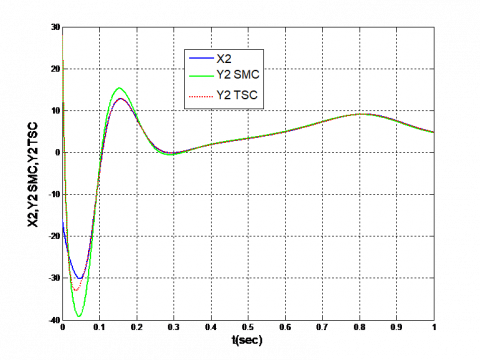## 23.png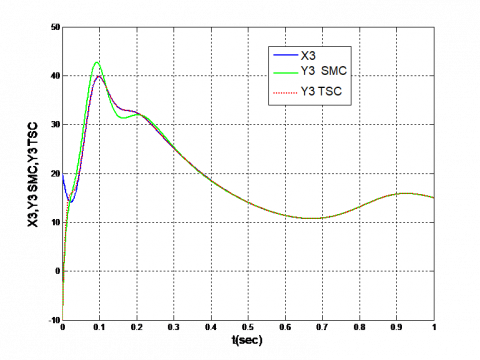## 24.png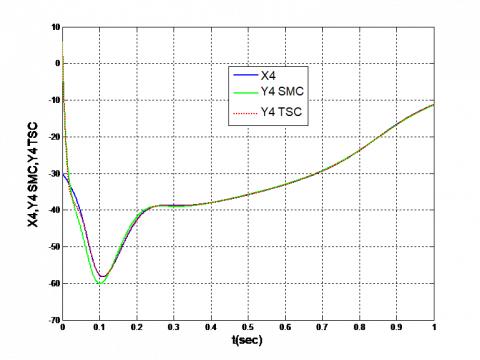Figure 2. Synchronization of the states with SMC and TSC

## 3.png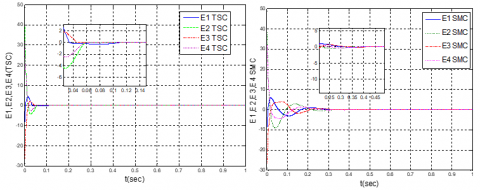Figure 3. Synchronization of the errors with SMC and TSC

## 41.png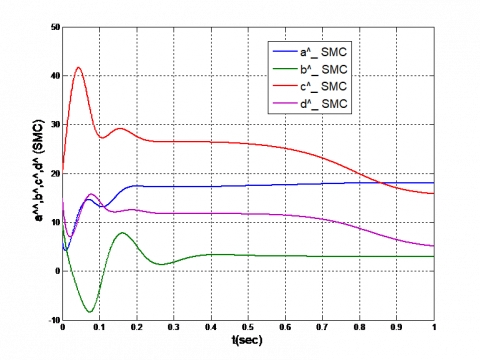## 42.png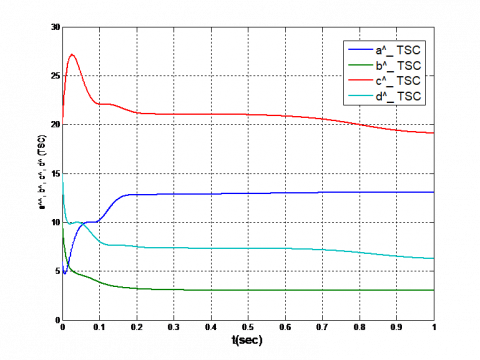Figure 4. Comparison of the parameter estimates $\hat{a}, \hat{b}, \hat{c}, \hat{d}$ between SMC and TSC

Table 1. Comparison of the response time of states between TSC and SMC

 Methods/response time of states TSC SMC x1,y1 0.031 sec 0.26 sec x2,y2 0.047 sec 0.17 sec x3,y3 0.046 sec 0.19 sec x4,y4 0.059 sec 0.18 sec

Table 2. Comparison of error response time between the two methods TSC and SMC

 Methods/response time of errors TSC SMC E1 0.127 0.44 E2 0.059 0.36 E3 0.048 0.45 E4 0.06 0.39

It is evident from Tables 1 and 2 that faster synchronization can be obtained using terminal synergetic control than with the use of SMC by a factor of 4. Sliding mode control induced errors subsides up to 0.4 seconds whereas those related to the proposed approach fade away within 0.15 seconds. So, thanks to the terminal synergetic control (TSC), the accuracy and the dynamic stability of the system is improved.

6. Conclusion

In this paper, an adaptive synergetic terminal approach has been proposed in a synchronization process of two hyperchaotic Zhou systems. Accordingly, the system model used was dismantled, then two identical hyperchaotic systems were synchronized using adaptive terminal synergetic control; provided that the system parameters are unknown. Finally, the findings were compared to sliding mode control results. The simulation outcomes show the prevalence of the synergetic approach over the sliding mode control. Indeed, two identical hyperchaotic systems have been synchronized using adaptive terminal synergetic control assuming unknown system parameters with good overall performance.

References

 Guessas, L., Abiza, M. (2018). Chaos suppression using genetically optimized PID control of the 4-D novel hyperchaotic vaidyanathan system. Engineering, Technology & Applied Science Research, 8(6): 3619-3623. https://doi.org/10.48084/etasr.2394

 Kocamaz, U.E., Uyaroğlu, Y., Kızmaz, H. (2017). Controlling hyperchaotic Rabinovich system with single state controllers: Comparison of linear feedback, sliding mode, and passive control methods. International Journal for Light and Electron Optics, 130: 914-921. https://doi.org/10.1016/j.ijleo.2016.11.006

 Rössler, O.E. (1979). An equation for hyperchaos. Physics Letters A, 71(2-3): 155-157. https://doi.org/10.1016/0375-9601(79)90150-6

 Jia, Q. (2007). Hyperchaos generated from the Lorenz chaotic system and its control. Physics Letters A, 366: 217-222. https://doi.org/10.1016/j.physleta.2007.02.024

 Chen, A., Lu, J., Lü, J., Yu, S. (2006). Generating hyperchaotic Lü attractor via state feedback control. Physica A, 364: 103-110. https://doi.org/10.1016/j.physa.2005.09.039

 Kapitaniak, T., Chua, L. (1994). Hyperchaotic attractors of undirectionally-coupled chua’s circuits. International Journal of Bifurcation and Chaos, 4(2): 477-482. https://doi.org/10.1142/S0218127494000356

 Qi, G.Y., van Wyk, M.A., Wyk, B.J., Chen, G.R. (2008). On a new hyperchaotic system. Physics Letters A, 372(2): 124-136. https://doi.org/10.1016/j.physleta.2007.10.082

 Dadras, S., Momeni, H.R., Qi, G., Wang, Z.L. (2012). Four-wing hyperchaotic attractor generated from a new 4D system with one equilibrium and its fractional-order form. Nonlinear Dynamics, 67: 1161-1173. https://doi.org/10.1007/S11071-011-0060-0

 Vaidyanathan, S., Azar, A.T., Rajagopal, K., Alexander, P. (2015). Design and SPICE implementation of a 12-term novel hyperchaotic system and its synchronization via active control. International Journal of Modeling, Identification and Control, 23(3): 267-277. https://doi.org/10.1504/IJMIC.2015.069936

 Sampath, S., Vaidyanathan, S., Volos, C.K., Pham, V.T. (2015). An eight-term novel four-scroll chaotic system with cubic nonlinearity and its circuit simulation. Journal of Engineering Science and Technology Review, 8(2): 1-6. https://doi.org/10.25103/jestr.082.01

 Pham, V.T., Vaidyanathan, S., Volos, C.K., Jafari, S., Wang, X. (2016). A chaotic hyperjerk system based on memristive device. Advances and Applications in Chaotic Systems, pp. 39-58. https://doi.org/10.1007/978-3-319-30279-9_2

 Smaoui, N., Karouma, A., Zribi, M. (2011). Secure communications based on the synchronization of the hyperchaotic Chen and the unified chaotic systems. Communications in Nonlinear Science and Numerical Simulation, 16(8): 3279-3293. https://doi.org/10.1016/j.cnsns.2010.10.023

 Wu, X.J., Wang, H., Lu, H.T. (2011). Hyperchaotic secure communication via generalized function projective synchronization. Nonlinear Analysis: Real World Applications, 12(2): 1288-1299. http://dx.doi.org/10.1016/j.nonrwa.2010.09.026

 Wu, X., Bai, C., Kan, H. (2014). A new color image cryptosystem via hyperchaos synchronization. Communications in Nonlinear Science and Numerical Simulation, 19(6): 1884-1897. http://10.1016j.cnsns.2013.10.025

 Zhang, Y., Wen, W., Su, M., Li, M. (2014). Cryptanalyzing a novel image fusion encryption algorithm based on DNA sequence operation and hyper-chaotic system. Optik - International Journal for Light and Electron Optics, 125(4): 1562-1564. http//doi:10.1016/j.ijleo.2013.09.018

 Liu, H., Wong, X., Kadir, A. (2013). Color image encryption using Choquet fuzzy integral and hyper chaotic system. Optik - International Journal for Light and Electron Optics, 124(18): 3527-3533. https://doi.org/10.1016/j.ijleo.2012.10.068

 Wei, X., Fang, Y.F., Li, Q. (2012). A novel four-wing hyper-chaotic system and its circuit implementation. Procedia Engineering, 29: 1264-1269. https://doi.org/10.1016/j.proeng.2012.01.124

 Niu, Y., Wang, X., Wang, M., Zhang, H. (2010). A new hyperchaotic system and its circuit implementation. Communications in Nonlinear Science and Numerical Simulation, 15(11): 3518-3524. https://doi.org/10.1016/j.cnsns.2009.12.005

 Pecora, L.M., Caroll, T.L. (1990). Synchronization in chaotic systems. Physical Review Letters, 64(8): 821-825. https://doi.org/10.1103/PhysRevLett.64.821

 Vaidyanathan, S., Karthikeyan, R. (2011). Anti-synchronization of hyperchaotic Lorenz and hyperchaotic Chen systems by adaptive control. International Journal of Signal System Control and Engineering Application, 4(2): 18-25. https://doi.org/10.3923/IJSSCEAPP.2011.18.25

 Vaidyanathan, S. (2015). Anti-synchronization of mathieu-van der pol chaotic systems via adaptive control method. International Journal of ChemTech Research, 8(11): 638-653.

 Vaidyanathan, S., Karthikeyan, R. (2012). Hybrid synchronization of hyperchaotic Lorenz and hyperchaotic Chen systems via active control. Journal of Engineering and Applied Sciences, 7: 254-264. https://doi.org/10.3923/JEASCI.2012.254.264

 Karthikeyan, R., Vaidyanathan, S. (2014). Hybrid chaos synchronization of four-scroll systems via active control. Journal of Electrical Engineering, 65(2): 97-103. https://doi.org/10.2478/jee-2014-0014

 Rosenblum, M.G., Picovisky, A.S., Kurths, J. (1997). From phase to lag synchronization in coupled chaotic oscillators. Physical Review Letters, 78: 4193. https://doi.org/10.1103/PhysRevLett.78.4193

 Rosenblum, M.G., Picovisky, A.S., Kurths, J. (1996). Phase synchronization of chaotic oscillators. Physical Review Letters, 76(11): 1804-1807. https://doi.org/10.1103/PhysRevLett.76.1804

 Astakhov, V., Shabunin, A., Anishchenko, V. (2000). Antiphase synchronization in symmetrically coupled self-oscillator. International Journal of Bifurcation and Chaos, 10(4): 849-857. https://doi.org/10.1142/S021812740000061X

 Rulkov, N.F., Sushchik, M.M., Tsimring, L.S., Ababarnel, H.D.I. (1995). Generalized synchronization of chaos in directionally coupled chaotic systems. Physical Review E, 51: 980-994. https://doi.org/10.1103/PhysRevE.51.980

 Mainieri, R., Rehacek, J. (1999). Projective synchronization in three dimensional chaotic system. Physical Review Letters, 82(15): 3042-3045. https://doi.org/10.1103/PhysRevLett.82.3042

 Sarasu, P., Vaidyanathan, S. (2011). The generalized projective synchronization of hyperchaotic Lorenz and hyperchaotic Qi systems via active control. International Journal of Soft Computing, 6: 216-223. https://doi.org/10.3923/IJSCOMP.2011.216.223

 Sarasu, P., Vaidyanathan, S. (2012). Adaptive controller design for the generalized projective synchronization of 4-scroll systems. International Journal of Signal System Control and Engineering Application, 5: 21-30. https://doi.org/10.39.23/IJSSCEAPP.2012.21.30

 Vaidyanathan, S., Pakiriswamy, S. (2013). Generalized projective synchronization of six-term Sundar Pandian chaotic systems by adaptive control. International Journal of Control Theory and Applications, 6(2): 153-163.

 Ho, M.C., Hung, Y.C. (2002) Synchronization of two different systems by using generalized active control. Physics Letters A, 301: 424-428. https://doi.org/10.1016/S0375-9601(02)00987-8

 Li, W., Liu, Z., Miao, J. (2010). Adaptive synchronization for a unified chaotic system with uncertainty. Communications in Nonlinear Science and Numerical Simulation, 15: 3015-3021. https://doi.org/10.1016/J.CNSNS.2009.11.002

 Chen, H.H., Sheu, G.J., Lin, Y.L., Chen, C.S. (2009). Chaos synchronization between two different chaotic systems via nonlinear feedback control. Nonlinear Analysis: Theory, Methods &Applications, 70: 4393-4401. https://doi.org/10.1016/J.NA.2008.10.069

 Tan, X., Zhang, J., Yang, Y. (2003). Synchronizing chaotic systems using backstepping design. Chaos, Solitons and Fractals, 16(1): 37-45. https://doi.org/10.10.16/S0960-0779(02)00153-4

 Vaidyanathan, S., Sivaperumal, S. (2013). Sliding controller design of hybrid synchronization of four-wing chaotic systems. International Journal of Soft Computing, 6: 224231. https://doi.org/10.39.23/IJSCOMP.2011.224.231

 Jiang, Z., Dougal, R.A. (2004). Synergetic control of power converters for pulse current charging of advanced batteries from a fuel cell power source. IEEE Trans. Power Electronics, 19: 1140-1150. https://doi.org/10.1109/TPEL.2004.830044

 Behih, K., Bouchama, Z., Saoudi, K. (2019). Finite-time fuzzy synergetic power system regulator. Soft Computing and Electrical Engineering, 1(1): 69-78.

 Nechadi, E. (2019). Adaptive fuzzy type-2 synergetic control based on bat optimization for multi-machine power system stabilizers. Engineering, Technology & Applied Science Research, 9(5): 4673-4678. https://doi.org/10.48084/etasr.2970

 Veselov, G., Sklyarov, A., Sklyarov, S., Semenov, V. (2016). Synergetic approach to the quadrotor helicopter control in an environment with external disturbances. International Siberian Conference on Control and Communications (SIBCON), Moscow, Russia. https://doi.org/10.1109/SIBCON.2016.7491680

 Zerroug, N., Harmas, M.N., Benaggoune, S., Bouchama, Z., Zehar, K. (2018). DSP-based implementation of fast terminal synergetic control for a DC-DC Buck converter. Journal of the Franklin Institute, 355: 2329-2343. https://doi.org/10.1016/J.JFRANKLIN.2018.01.004

 Bouchama, Z., Harmas, M.N., Zehar, K. (2019). Finite time nonlinear control of a DC-DC Buck converter. Soft Computing and Electrical Engineering, 1(1): 36-45.

 Zhou, P., Cao, Y.X., Cheng, X.F. (2009). A new hyperchaos system and its circuit simulation by EWB. Chinese Physics B, 18(4): 1394-1398. https://doi.org/10.1088/1674-1056/18/4/018